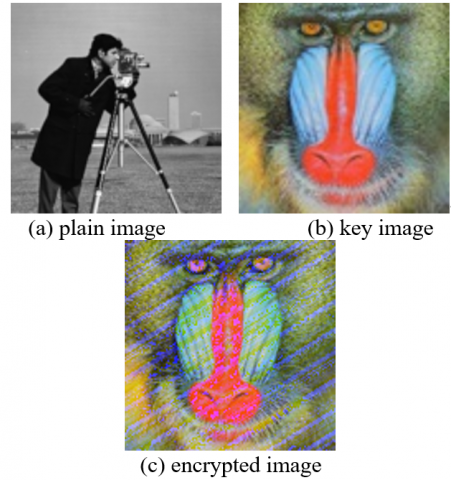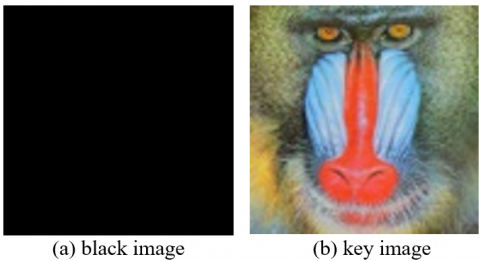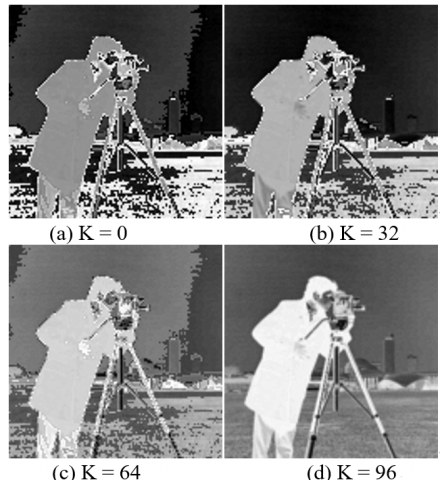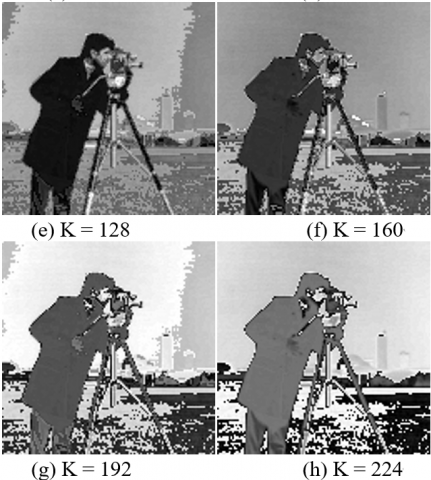# Cryptanalysis of a Pixel Permutation Based Image Encryption Technique Using Chaotic Map

Cryptanalysis of a Pixel Permutation Based Image Encryption Technique Using Chaotic Map
Laboratory of Power Electronics and Industrial Control, Department of Electronics, Faculty of Science and Technology, University of Setif-1, Setif 19000, Algeria

Corresponding Author Email:
abdelaziz.mokhnache@univ-setif.dz
Page:
95-100
|
DOI:
https://doi.org/10.18280/ts.370112
23 November 2019
|
Accepted:
10 January 2020
|
Published:
29 February 2020
| Citation

OPEN ACCESS

Abstract:

Recently, a new image encryption algorithm was proposed by Anwar and Meghana. This encryption scheme uses the Arnold's chaotic cat map to permute the image pixels. The resulting image is then confused with both a secret image provided as part of the secret key and a secret value selected randomly from the permuted image. Using a random image as part of the secret key, gives this algorithm an infinite key space which increases its efficiency against brute-force attacks. In order to help improving the security of modern image encryption schemes, this paper presents a cryptanalysis of the proposed algorithm using a combination of chosen-plaintext and brute-force attacks. First, the infinite key space that the secret image offers is broken using a chosen-plaintext attack. Then, the permutation phase is reversed through a series of chosen-plaintext attacks too. Finally, the secret value chosen randomly from the permuted image is easily brute-forced due to its reduced number of possible values. By applying the above method, it is possible to restore the plain version of any image that was encrypted using the former encryption algorithm. Thus, relying on this algorithm to encrypt real sensitive data is not secure.

Keywords:

chaos, chosen-plaintext attack, brute-force attack, image encryption

1. Introduction

Nowadays, the use of social networks and the means of communication became part of almost everybody's daily life. Images are one of the most used forms of information on those platforms. A lot of sent and shared images contain personal and sensitive information that should be kept private. Encryption is one of the solutions proposed for the privacy of these data.

But unlike textual information, images have specific particularities like the redundancy of pixels, the strong correlation between them as well as their important size . This makes the conventional encryption schemes such as Rivest-Shamir-Adleman algorithm (RSA), Data Encryption Standard (DES) and Advanced Encryption Standard (AES) not suitable for image encryption .

In the recent years, researchers have developed many image encryption schemes based on different theories like: chaos [3-8], Deoxyribonucleic Acid (DNA) [9-12] and Substitution-Box (S-box) [13-15]. These schemes were designed to solve different problems. However, many cryptanalysis works have been published on the other hand, and many of the proposed algorithms were found unsecure to some types of attacks.

A simple and efficient image encryption scheme was proposed by Çavuşoğlu et al.  using an S-Box generated by a Random Number Generator (RNG) based on a new chaotic system. The proposed RNG was evaluated against the National Institute of Standards and Technology randomness test (NIST-800-22) and it gave good results. Compared to AES, the proposed algorithm has a larger key space that makes brute-force attacks harder. But, Zhu et al.  proved that this algorithm has some undiscovered security flaws. They were able to totally break it using a chosen-plaintext attack where only two chosen plain-images were required.

Another algorithm based on a chaotic map and information entropy was proposed by Ye et al. . This algorithm deviated a bit from Fridrich’s well known permutation-diffusion structure by inserting a modulation phase between the two phases. In addition, information entropy was used to influence the generation of the keystream. Experimental results demonstrated a good performance and security. Hence, the authors claimed that the proposed algorithm can be used as a secure and effective communication method for images. However, Li et al.  reported some security defects of the chaos-based pseudo-random number generator used in this algorithm. Furthermore, they were able to break its one round version completely with a differential attack.

Based on the security analysis of the pure Chaotic Tent Map (CTM) based scheme, Wu et al.  proposed a novel image encryption algorithm by using the combination of the rectangular transform and the CTM principle. They have also improved the key sensitivity by generating the key streams based on both the secret keys and the plain image. Although the remarkable improvements over the pure CTM-based schemes, this scheme was broken by Zhu et al.  using chosen-plaintext attacks. They were able to break the equivalent keys of the cryptosystem and decode the target cipher image successfully.

A novel image cryptosystem was proposed based on a two dimensional modified henon map (2D-MHM) and sine map . The algorithm employs confusion and diffusion operations in consecutive manner which is different from traditional chaos-based cryptosystems. Hybrid chaotic shift transform (HCST) was introduced to perform confusion operation which is controlled by 2D-MHM. Simulation results demonstrated that the proposed image cryptosystem was able to resist various cryptanalytic attacks. However, Zhou et al. found it insecure against the chosen-plaintext attack and proposed a method to break it using several chosen-plaintext attacks .

From the above discussion, we see clearly that cryptanalysis works are so important because they help revealing vulnerabilities in encryption schemes and facilitate the development of cryptography in general . They can also prevent unsafe encryption algorithms from spreading and being applied to actual communications with real sensitive data.

When a study proposing a new encryption algorithm is published, it should be a subject for other studies that evaluate the proposed algorithm’s security and robustness against known attacks. These studies must be conducted on every encryption scheme in order to verify the security claims announced by the original authors and evaluate the safety level that this scheme offers when it is used to encrypt real data.

Recently, a new image encryption algorithm based on a pixel permutation technique using chaotic map was proposed by Anwar and Meghana . This encryption scheme uses a modified version of the Arnold's chaotic cat map to permute the image pixels. The resulting image is then confused with a secret value selected randomly from the permuted image. Finally, the output image is hidden in another image selected as part of the secret key. Given that this key image can be any random image existing in the world, this algorithm has an infinite key space which increases its efficiency against brute-force attacks. Thus, it is supposed to be secure for real life applications.

An attentive investigation reveals that the former algorithm has some serious vulnerabilities that make it completely broken and unsecure to be used with real data. But no study has covered this topic until now.

This paper presents a cryptanalysis of the former encryption scheme. The rest of it is organized as follows. In Section 2 the algorithm under study is described briefly. Detailed cryptanalysis of the proposed algorithm is presented in Section 3. The cryptanalysis experimental results are given in Section 4. Finally, some concluding remarks are given in Section 5.

2. Encryption Algorithm Description

A new image encryption algorithm based on Arnold’s cat map was proposed . The novelty in this algorithm was the use of a random image as part of the secret key. Unlike other existing encryption algorithms that transform images to a noise like image, this one transforms the plain image into a visually meaningful encrypted one which may reduce the number of attacks on the image.

The proposed algorithm is based on pixel permutation using a variation of the Arnold’s chaotic cat map algorithm, where each pixel is replaced by another pixel from the image. The pixel permutation is performed as shown in Eq. (1).

$\left[\begin{array}{c}x_{i} \\ y_{i}\end{array}\right]=\left[\begin{array}{cc}1 & \varepsilon \\ \varphi & \varphi \varepsilon+1\end{array}\right]\left[\begin{array}{c}x_{i-1} \\ y_{i-1}\end{array}\right]$   (1)

This process is executed T times $(T \geq 1)$. The other parameters $\mathcal{E}$ and $\varphi$ are chosen such that the matrix determinant is equal to 1.

The obtained image is normalized. From that normalized image N, a random pixel is chosen to use its value a secret key. Then, a new matrix having the same image dimensions and initialized by K is created, $P(i, j)=K$.

To obtain a semi-encrypted image Z, a bit wise XOR  is then performed between the normalized image and the newly created matrix P. This semi-encrypted image is then masked into a general image $K_{R e f}$, called the key image, to elude the attackers.

\begin{aligned} Z(i, j) &=N(i, j) \oplus P(i, j) \\ E(i, j) &=K_{R e f}(i, j)-Z(i, j) \end{aligned}   (2)

Theoretically, the proposed algorithm has an infinite key space because any random image can be chosen as part of the secret key. Thus, it is very efficient against brute-force attacks aiming to decode the encrypted image by an exhaustive searching for the possible choices in the key space.

Because of the infinite key space and changing both pixels’ values and locations during the encryption process, the authors claimed that this algorithm is resistant to known attacks and has a high level of security.

3. Cryptanalysis of the Proposed Algorithm

An attentive investigation of the proposed algorithm reveals some security issues. Those found vulnerabilities will be exploited to break this cryptosystem and decode the encrypted image using a combination of chosen-plaintext and brute-force attacks.

3.1 Revealing the key image

The strongest part of this algorithm is the use of a key image that could be any random image that exists. This allows the algorithm to have an infinite key-space. This can be true in theory. But in reality, the key image is easily identifiable when looking to the cipher image. Actually, they are nearly the same in most cases. This reveals some information about the used key and this should not happen in cryptosystems.  In the case when the key image is an image that is available online, it will be just a reverse image search away.

Even in cases where the key image can’t be looked up online, the algorithm is not taking full advantage of the large key space that the key image offers. It may be able to resist brute-force attacks, but it is weak against chosen-plaintext attacks. The exact key image can be restored completely using a single chosen plain-image.

According to the algorithm, if a totally black image is encrypted, the result will be the exact key image as shown below.

$K_{R e f}=$ encrypt $(\text {zeros}(m, n))$   (3)

In the encryption process, the permutation phase is useless toward totally black images because all pixels’ values are equal. The secret key chosen as a random pixel’s value of the permuted image will be equal to 0 because all pixels’ values of the images are zeros. Hence, the diffusion phase will have no effect too because 0 is a neutral element in the XOR operation. In this case, the encrypted image will be the result of subtracting a black image from the key image. Therefore, the encrypted image will be equal to the exact key image provided as a secret key to the encryption algorithm.

Since the key image $K_{R e f}$ is already revealed from the previous step, it is possible to unmask the semi-encrypted image $Z(i, j)$ from the encrypted one $E(i, j)$ with a simple subtraction as shown in Eq. (4) below.

$Z(i, j)=K_{R e f}(i, j)-E(i, j)$   (4)

3.3 Reversing the permutation phase

The permutation phase in the proposed algorithm is based on Arnold’s cat map. Without knowing its parameters and the number of times it has been executed, it is hard to reverse it using a brute force attack. However, no matter what parameters are used and how many times the permutation operation is executed, it is possible to model that whole process using a permutation vector PV on the reshaped 1D version of the image.

\begin{aligned} I &=reshape(I, 1, m \times n) \\ I(i) &=I(P V(i)) \\ I &=reshape (I, m, n) \end{aligned}   (5)

In this model, if the permutation vector is known, the permutation process can be easily reversed. Actually, using a series of chosen-plaintext attacks, the exact permutation vector that was used in the encryption process can be restored completely.

Given an image $I_{0}$ of size $(m \times n)$ chosen such that the pixel $I_{0}(0,0)=255$ and zeros elsewhere. $E_{0}$ is noted as the encrypted image corresponding to the plain image $I_{0}$. Knowing both the key image $K_{R e f}$ and the encrypted image $E_{0}$, the semi-encrypted image $Z_{0}$ corresponding to $I_{0}$ can be extracted using Eq. (4).

We know from Eq. (2) that $Z_{0}=N_{0} \oplus P_{0}$. In the actual case, there will be just two possible values of $P_{0}$, $P_{0}(i, j) \in\{0,255\}$ because it is randomly selected from the plain image $I_{0}$. Both of these values are neutral elements for the XOR operation. Therefore, the diffusion phase will have no effect on the permuted image and we will get $N_{0}=Z_{0}$.

Now, the attacker has the plain image $I_{0}$ and the corresponding permuted image $N_{0}$. The permutation positions $i_{0}$ (the index of 255 inside $I_{0}$) and $i_{0}^{\prime}$ (the index of 255 inside $N_{0}$) can be determined using a simple search. Thus, the first element of the permutation vector is found.

\begin{aligned} I_{0}=reshape\left(I_{0}, 1, m \times n\right)\\N_{0}=reshape\left(N_{0}, 1, m \times n\right) \\ i_{0}= indexOf\left(255, I_{0}\right) \\ i_{0}^{\prime}=indexOf\left(255, N_{0}\right) \\ P V\left(i_{0}\right) = i_{0}^{\prime} \end{aligned}      (6)

The other permutation vector elements can be restored by repeating this process $(m \times n)$ times using different images $I_{i x j}$ each time, where the pixel $I_{i \times j}(i, j)=255$ and zeros elsewhere, $i \in\{0, \ldots, m\}$ and $j \in\{0, \ldots, n\}$.

Once the permutation vector is restored, the permutation phase can be reversed as shown below.

\begin{aligned} I =r e s h a p e(I, 1, m \times n) \\ I(P V(i))= I(i) \\ I=r e s h a p e(I, m, n) \end{aligned}  (7)

3.4 Brute forcing the encryption key

At this stage, revealing the original image is just one step ahead. Knowing the semi-encrypted image Z and the permutation vector from the steps above, the permutation phase can be reversed. The result is the XOR output of the plain image and the secret key.

$I \oplus P=reversePermutation(Z)$  (8)

Although $I \oplus P$ is not the exact original image, it can be visually identifiable if it is a well-known image. This can be sufficient in some cases where the attacker doesn’t care a lot about image’s details.

Because the secret key is a random pixel value from the permuted image, it has a small key-space of just 256 possible values which are easy to brute-force. By iterating over the 256 possible values of the secret key P, 256 samples will be generated. One of those samples is the exact original image that was encrypted with the algorithm. The other samples are more or less similar to the original one. Depending on the details' importance for the attacker, the number of samples can be reduced.

If the secret key value happens to be 2 or 255, the diffusion phase will not have any effect. There will be no need for this step at all because the previous step will output the exact original image. This situation is more likely to happen when encrypting images containing a lot of black or white pixels.

4. Results and Discussion

To verify the effectiveness of the proposed cryptanalysis, some experiments have been executed on the grayscale image “Cameraman” of size $256 \times 256$ as shown in Figure 1(a). This image is encrypted with the algorithm  using “baboon” image as the key image shown in Figure 1(b). The corresponding cipher image is shown in Figure 1(c).

In our example, we’ve chosen to mask the semi-encrypted image inside the blue channel of the RGB key image.

## 1.pngFigure 1. Image encryption using the proposed algorithm

To recover the key image, a totally black image shown in Figure 2(a) is encrypted using the previous encryption process. Clearly, the corresponding encrypted image shown in Figure 2(b) is the Key Image used as a secret key by the encryption algorithm.

## 2.pngFigure 2. Revealing the key image using a chosen-plaintext attack

Because the used key image in this example is a well-known image that could be found online (baboon), we tried a reverse image search using Google search engine. Because of the strong similarity between the key image and the encrypted one, Google reverse image search was able to guess the original image perfectly. The result is shown in Figure 3. In this case, the search result image can be downloaded and used to recover the original plain image following the rest of the steps mentioned above.

Sure, this method is not going to work perfectly in all cases. It just works when the exact key image is available online and a big level of similarity exists between it and the resulting cipher image. The previous method is always preferred over this one.

## 3.jpgFigure 3. Revealing the key image using an online reverse image search using Google

The Figure 4(a) shows the semi-encrypted image after its extraction from the encrypted one. After reversing the permutation phase, the image shown in Figure 4(b) is obtained. This image is the result of the XOR operation between the plain image and the secret value K which is a random pixel value chosen from the permuted image. Although it is not the exact same plain image that have been encrypted, it is clear enough to identify it and know for sure that it represents the "Cameraman" image.

Because "Cameramen" is a well-known test image, it was easy to guess without going much further. But, if the encrypted image was for a less known image or contains some text, it won't be easy to exploit in this form. For that reason, brute-forcing the Key will be required.

## 4.pngFigure 4. Image cryptanalysis using the proposed method

To recover the original image in a pixel perfect format, trying the 256 different cases of the key is required. For illustration purposes, just 8 samples are going to be generated as shown in Figure 5. The corresponding key values that will be used are $K \in\{0,32,64,96,128,160,192,224\}$.

## 5a.png## 5b.pngFigure 5. Brute-forcing the encryption key

TABLEFigure 5(e) where k=128 represents the most accurate generated sample compared to the original image. This means that the exact key value is not too far from 128. If more details are needed, the attaquer can easilly investigate the other values and generate all the 256 possible values.

5. Conclusions

This paper attacks an image encryption algorithm which was recently proposed by Anwar and Meghana  and announced to be secure for real data encryption. Following are some of the most important weaknesses identified in the proposed algorithm that made it totally breakable:

• In this encryption scheme, the cipher image has a lot of similarities with the key image. In other words, the cipher image leaks information about the secret key, which is a vulnerability that should never exist in a cryptosystem.
• Making multiple permutation only rounds doesn’t offer any further improvements to the algorithm security. Any number of permutation rounds can be modeled with a single permutation vector at the end.
• Because the permutation key is chosen as a random pixel value from the permuted image, it has a reduced key space that can be brute-forced easily. Another issue with this approach is the possibility of the random pixel value to be 0 or 255. Thus, the diffusion phase will have no effect at all.
• Having just one round of encryption and a weak dependency on the plain image creates some linearity between the plain and the encrypted image, which is a sign of an unsecure algorithm.

After an analysis exploiting the above vulnerabilities, the proposed algorithm is found to be weak against some well-known types of attacks, namely chosen-plaintext and brute-force attacks. Our research and experimental results proved the claim that this encryption technique can be used to securely share information in form of images to be false. Images encrypted using this scheme can be decoded using chosen plaintext attacks. Therefore, relying on this cryptosystem to encrypt images containing sensitive information is not secure.

References

 Li, S., Chen, G., Cheung, A., Bhargava, B., Lo, K.T. (2007). On the design of perceptual MPEG-Video encryption algorithms. IEEE Transactions on Circuits and Systems for Video Technology, 17(2): 214-223. https://doi.org/10.1109/TCSVT.2006.888840

 Wang, X.Y., Yang, L., Liu, R., Kadir, A. (2010). A chaotic image encryption algorithm based on perceptron model. Nonlinear Dynamics, 62(3): 615-621. https://doi.org/10.1007/s11071-010-9749-8

 Bashir, Z., Wątróbski, J., Rashid, T., Zafar, S., Sałabun, W. (2017). Chaotic dynamical state variables selection procedure based image encryption scheme. Symmetry, 9(12): 312. https://doi.org/10.3390/sym9120312

 Parvaz, R., Zarebnia, M. (2018). A combination chaotic system and application in color image encryption. Optics & Laser Technology, 101: 30-41. https://doi.org/10.1016/j.optlastec.2017.10.024

 Herbadji, D., Derouiche, N., Belmeguenai, A., Herbadji, A., Boumerdassi, S. (2019). A tweakable image encryption algorithm using an improved logistic chaotic map. Traitement du Signal, 36(5): 407-417. https://doi.org/10.18280/ts.360505

 Cai, Q. (2019). A secure image encryption algorithm based on composite chaos theory. Traitement du Signal, 36(1): 31-36. https://doi.org/10.18280/ts.360104

 Wang, X.Y., Zhang, J.J., Cao, G.H. (2019). An image encryption algorithm based on ZigZag transform and LL compound chaotic system. Optics & Laser Technology, 119: 105581. https://doi.org/10.1016/j.optlastec.2019.105581

 Hua, Z., Zhou, Y., Huang, H. (2019). Cosine-transform-based chaotic system for image encryption. Information Sciences, 480: 403-419. https://doi.org/10.1016/j.ins.2018.12.048

 Wu, J., Liao, X., Yang, B. (2018). Image encryption using 2D Hénon-Sine map and DNA approach. Signal Processing, 153: 11–23. https://doi.org/10.1016/j.sigpro.2018.06.008

 Huo, D., Zhou, D., Yuan, S., Yi, S., Zhang, L., Zhou, X. (2019). Image encryption using exclusive-OR with DNA complementary rules and double random phase encoding. Physics Letters A, 383(9): 915-922. https://doi.org/10.1016/j.physleta.2018.12.011

 Chai, X., Fu, X., Gan, Z., Lu, Y., Chen, Y. (2019). A color image cryptosystem based on dynamic DNA encryption and chaos. Signal Processing, 155: 44-62. https://doi.org/10.1016/j.sigpro.2018.09.029

 Yang, Y.G., Guan, B.W., Li, J., Li, D., Zhou, Y.H., Shi, W.M. (2019). Image compression-encryption scheme based on fractional order hyper-chaotic systems combined with 2D compressed sensing and DNA encoding. Optics & Laser Technology, 119: 105661. https://doi.org/10.1016/j.optlastec.2019.105661

 Liu, H., Kadir, A., Niu, Y. (2014). Chaos-based color image block encryption scheme using S-box. AEU - International Journal of Electronics and Communications, 68(7): 676-686. https://doi.org/10.1016/j.aeue.2014.02.002

 Liu, H., Kadir, A., Sun, X., Li, Y. (2018). Chaos based adaptive double-image encryption scheme using hash function and S-boxes. Multimedia Tools and Applications, 77(1): 1391-1407. https://doi.org/10.1007/s11042-016-4288-z

 Silva-García, V.M., Flores-Carapia, R., Rentería-Márquez, C., Luna-Benoso, B., Aldape-Pérez, M. (2018). Substitution box generation using Chaos: An image encryption application. Applied Mathematics and Computation, 332: 123-135. https://doi.org/10.1016/j.amc.2018.03.019

 Çavuşoğlu, Ü., Kaçar, S., Pehlivan, I., Zengin, A. (2017). Secure image encryption algorithm design using a novel chaos based S-Box. Chaos, Solitons & Fractals, 95: 92-101. https://doi.org/10.1016/j.chaos.2016.12.018

 Zhu, C., Wang, G., Sun, K. (2018). Cryptanalysis and improvement on an image encryption algorithm design using a novel chaos based S-Box. Symmetry. 10(9): 399. https://doi.org/10.3390/sym10090399

 Ye, G., Pan, C., Huang, X., Zhao, Z., He, J. (2018). A chaotic image encryption algorithm based on information entropy. International Journal of Bifurcation and Chaos, 28(1): 1850010. https://doi.org/10.1142/S0218127418500104

 Li, C., Lin, D., Feng, B., Lü, J., Hao, F. (2018). Cryptanalysis of a chaotic image encryption algorithm based on information entropy. IEEE Access, 675834-75842. https://doi.org/10.1109/ACCESS.2018.2883690

 Wu, X., Zhu, B., Hu, Y., Ran, Y. (2017). A novel color image encryption scheme using rectangular transform-enhanced chaotic tent maps. IEEE Access, 56429-6436 https://doi.org/10.1109/ACCESS.2017.2692043

 Zhu, C., Sun, K. (2018). Cryptanalyzing and improving a novel color image encryption algorithm using RT-enhanced chaotic tent maps. IEEE Access, 618759-18770. https://doi.org/10.1109/ACCESS.2018.2817600

 Sheela, S.J., Suresh, K.V., Tandur, D. (2018). Image encryption based on modified Henon map using hybrid chaotic shift transform. Multimedia Tools and Applications, 77(19): 25223-25251. https://doi.org/10.1007/s11042-018-5782-2

 Zhou, K., Xu, M., Luo, J., Fan, H., Li, M. (2019). Cryptanalyzing an image encryption based on a modified Henon map using hybrid chaotic shift transform. Digital Signal Processing, 93: 115-127. https://doi.org/10.1016/j.dsp.2019.07.013

 Arroyo, D., Alvarez, G., Li, S. (2009). Some hints for the design of digital chaos-based cryptosystems: lessons learned from cryptanalysis. IFAC Proceedings Volumes, 42(7): 171-175. https://doi.org/10.3182/20090622-3-UK-3004.00034

 Anwar, S., Meghana, S. (2019). A pixel permutation based image encryption technique using chaotic map. Multimedia Tools and Applications, 78(19): 27569-27590. https://doi.org/10.1007/s11042-019-07852-2